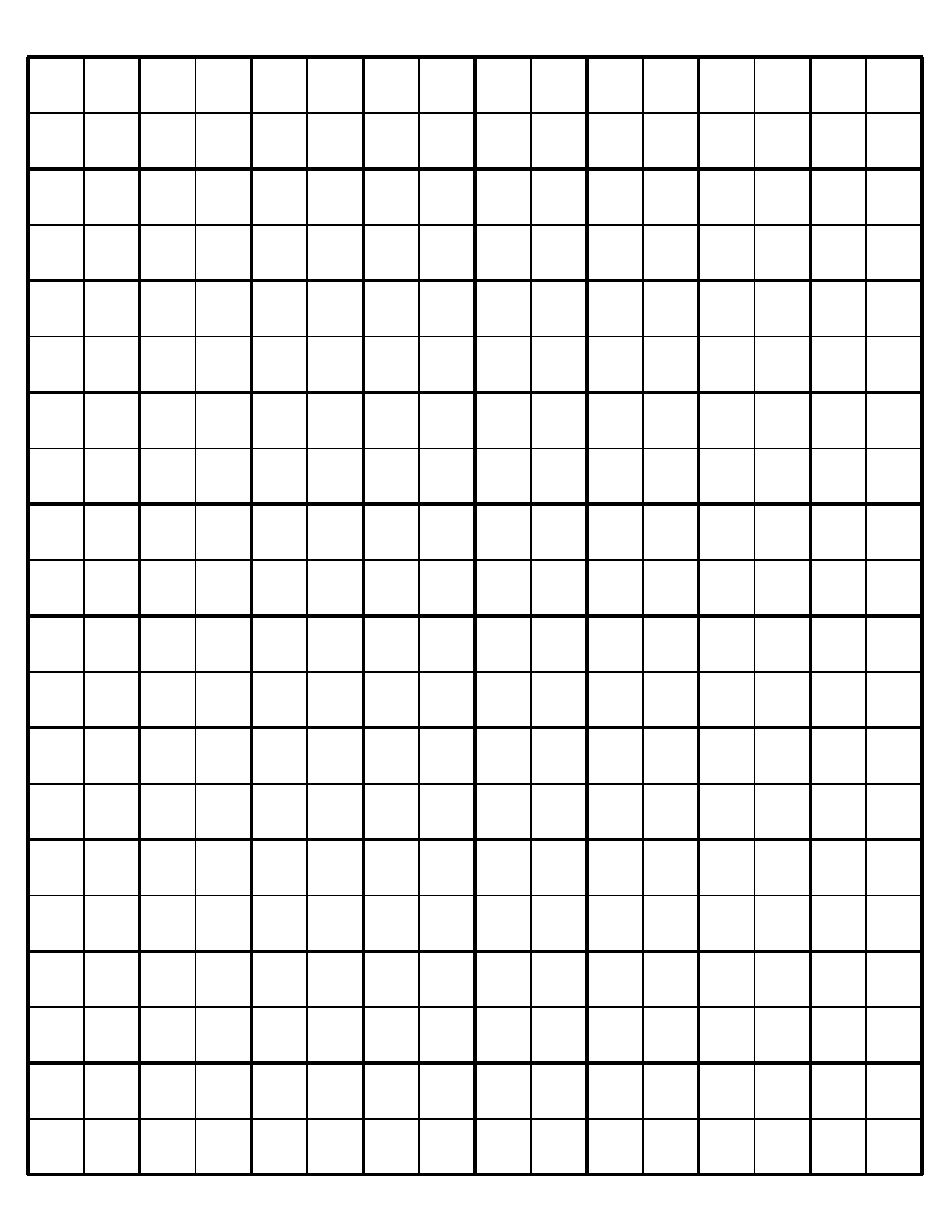# Black and White Math Graph Paper

Black and white math graph paper is commonly used for plotting mathematical functions, creating charts and graphs, and solving geometric problems. It provides a structured grid system that helps with organizing data and visualizing mathematical concepts.

## FAQ

Q: What is black and white math graph paper?
A: Black and white math graph paper is a type of paper that has a grid of squares printed on it. The squares are usually used for graphing or solving math problems.

Q: How is black and white math graph paper different from regular paper?
A: Black and white math graph paper has a grid of squares, while regular paper does not. The grid helps in drawing graphs or solving math problems.

Q: What are some uses of black and white math graph paper?
A: Black and white math graph paper is commonly used for graphing functions, plotting coordinates, solving equations, and doing geometry or trigonometry problems.

•